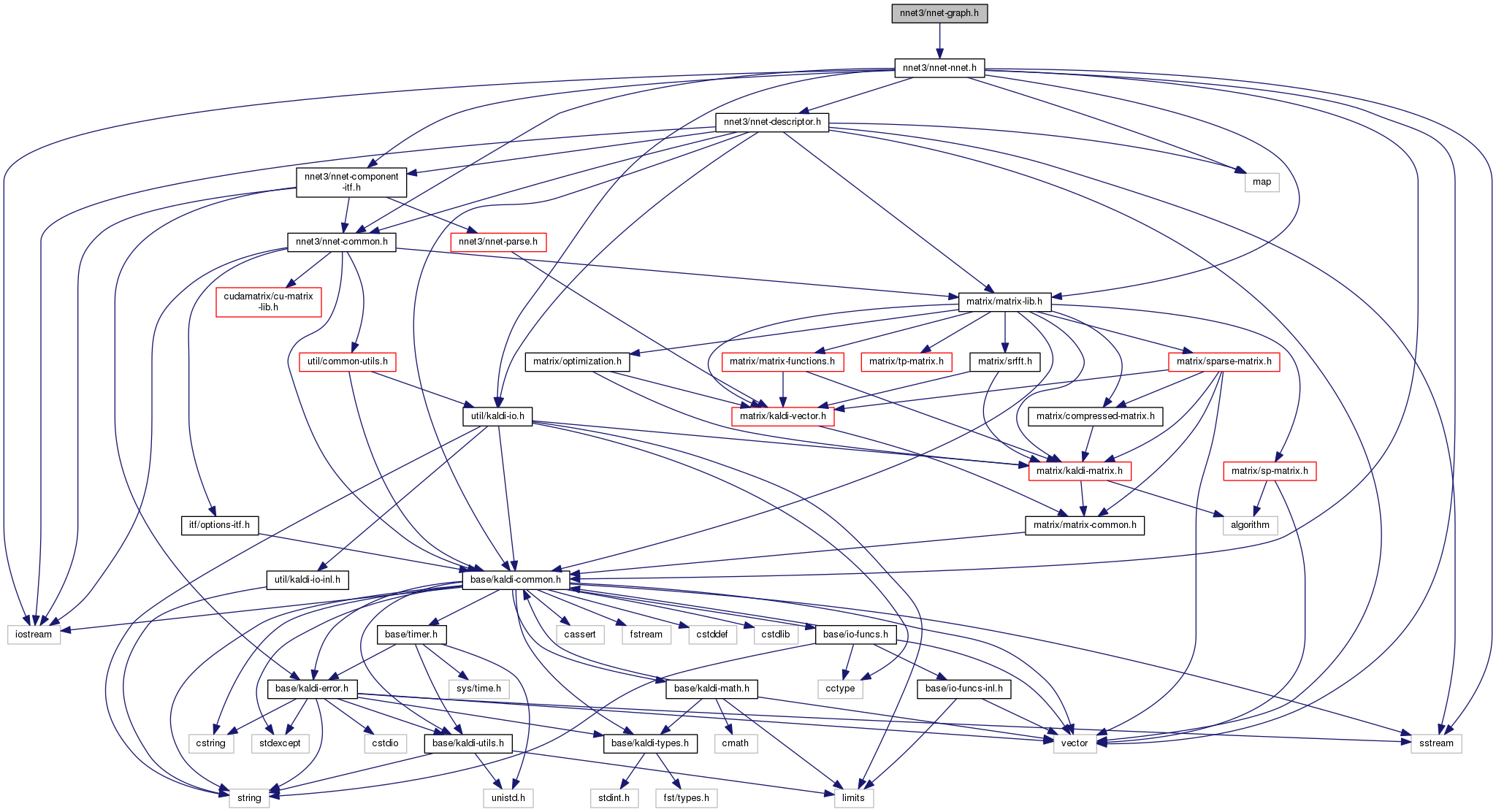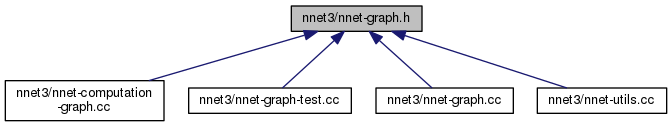nnet-graph.h File Reference

This file contains a few functions that treat the neural net as a graph on nodes: e.g. More...

`#include "nnet3/nnet-nnet.h"`
Include dependency graph for nnet-graph.h:This graph shows which files directly or indirectly include this file:Go to the source code of this file.

## Namespaces

kaldi
This code computes Goodness of Pronunciation (GOP) and extracts phone-level pronunciation feature for mispronunciations detection tasks, the reference:

kaldi::nnet3

## Functions

std::string PrintGraphToString (const std::vector< std::vector< int32 > > &graph)
Prints a graph to a string in a pretty way for human readability, e.g. More...

void NnetToDirectedGraph (const Nnet &nnet, std::vector< std::vector< int32 > > *graph)
This function takes an nnet and turns it to a directed graph on nodes. More...

void FindSccs (const std::vector< std::vector< int32 > > &graph, std::vector< std::vector< int32 > > *sccs)
Given a directed graph (where each std::vector<int32> is a list of destination-nodes of arcs coming from the current node), partition it into strongly connected components (i.e. More...

bool GraphHasCycles (const std::vector< std::vector< int32 > > &graph)
This function returns 'true' if the graph represented in 'graph' contains cycles (including cycles where a single node has an arc to itself). More...

void MakeSccGraph (const std::vector< std::vector< int32 > > &graph, const std::vector< std::vector< int32 > > &sccs, std::vector< std::vector< int32 > > *scc_graph)
Given a list of sccs of a graph (e.g. More...

void ComputeTopSortOrder (const std::vector< std::vector< int32 > > &graph, std::vector< int32 > *node_to_order)
Given an acyclic graph (where each std::vector<int32> is a list of destination-nodes of arcs coming from the current node), compute a topological ordering of the graph nodes. More...

void ComputeGraphTranspose (const std::vector< std::vector< int32 > > &graph, std::vector< std::vector< int32 > > *graph_transpose)
Outputs a graph in which the order of arcs is reversed. More...

void ComputeNnetComputationEpochs (const Nnet &nnet, std::vector< int32 > *node_to_epoch)
This function computes the order in which we need to compute each node in the graph, where each node-index n maps to an epoch-index t = 0, 1, ... More...

## Detailed Description

This file contains a few functions that treat the neural net as a graph on nodes: e.g.

to find strongly-connected components in the graph, and from there to compute an ordering on the graph nodes.

Definition in file nnet-graph.h.